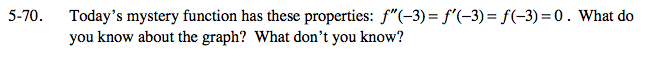### Home > CALC > Chapter 5 > Lesson 5.2.2 > Problem5-70

5-70.When a 1st-derivative is equal to zero at x = a, then x = a is a CANDIDATE for a local max or a local min. When a 2nd-derivative is equal to zero at x = a, then x = a is also a CANDIDATE for a point of inflection. Obviously, the candidate cannot hold all three positions... so, how do we determine if x = a is a local max, a local min or a POI?